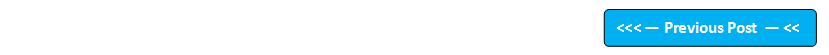## Blog Pages

### 167. Understanding API (Application Programming Interface)In order to understand JAVA completely, you need to understand its API first.  API stands for Application Programming Interface.

What is an API ?

An API is a set of predefined commands, functions and protocols which  programmers use while developing the code.

What are predefined functions ?

Instead of writing the code from scratch we can use the predefined methods.

Lets understand the difference between writing the code from scratch and using predefined methods by implementing a java program to print the greater of two numbers.

Lets implement this on Eclipse IDE -

1. Launch Eclipse IDE, create a new Java Project 'Project 014' as shown below -

2. Create a package 'package_014' under the Java Project 'Project 014' as shown below -

3. Create a Class 'Class014' with main( ) method under the package 'package_014' as shown below -

4. Now lets write the code from scratch to print the greater of two numbers as shown below -

5. Instead of writing the above code from scratch, we can use a predefined function max( )  in Java API to find the greater of two number. In order to use the max( ) method we have to call it from its Class called Math. Math is a static class, so there is no need of creating objects for calling the methods inside it. So we can call max( ) method in Math Class just by specifying Math.max( ) in the code as shown below -

Hence its very clear that by using Java API, we can use its predefined functions to avoid writing the code from the scratch.